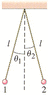# Spheres and electrostatics

physicsss
the below figure make small angles theta1 and theta2 with the vertical.

Figure 21-50
(a) If Q1 = Q, Q2 = 3Q, and m1 = m2 = m, determine the ratio theta1 / theta2.

(b) If Q1 = Q, Q2 = 2Q, and m1 = m, and m2 = 4m, determine the ratio of theta 1 / theta 2.

I don't get the forces that are acting on the spheres. I know there's gravitational, electrostatic, and tensional forces. How do I start the problem?

#### Attachments

•123.gif
4.3 KB · Views: 451

FluxCapacitator
The number one thing you have to realize is that the balls are in equilibrium, or, the forces in all directions cancel out.

You have weight vectors pointing down, electrostatic force vectors pointing left and right, and Tension vectors pointing up diagonally. The forces have to cancel out, so just make equations in the horizontal and vertical directions, like $$\sum F_{x}=0 , \sum F_{y}=0$$.

Hope I helped you out.

physicsss
Don't the electrostatic forces cancel out though since they are the same magnitude and opposite direction?

If that's the case, wouldn't that make tension of the two ropes be the same, and the answer to (a) would just be 1?

Last edited:
physicsss
anyone got an idea?

Nylex
physicsss said:
Don't the electrostatic forces cancel out though since they are the same magnitude and opposite direction?

If that's the case, wouldn't that make tension of the two ropes be the same, and the answer to (a) would just be 1?

The electrostatic forces are the same magnitude and in opposite directions, but they're acting on two different charges! Each charge is in equilibrium and the electrostatic force is balanced by a component of the tension in the string. The tension in each string is not the same, because of the weight of the balls.

physicsss
But for part a), the weight of the balls are the same.